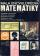Stairs

Between adjacent levels are 15 stairs. If step will be 1.2 cm lower there would be 16 stairs. What is the stair height?

Result

x =  19.2 cm

Solution:

15x = 16(x-1.2)

x = 19.2

x = 965 = 19.2

Calculated by our simple equation calculator.

Leave us a comment of this math problem and its solution (i.e. if it is still somewhat unclear...):Be the first to comment!To solve this verbal math problem are needed these knowledge from mathematics:

Do you have a linear equation or system of equations and looking for its solution? Or do you have quadratic equation? Do you want to convert length units?

Next similar examples:

1. NormThree workers planted 3555 seedlings of tomatoes in one dey. First worked at the standard norm, the second planted 120 seedlings more and the third 135 seedlings more than the first worker. How many seedlings were standard norm?
2. Tickets 3A total of 645 tickets were sold for the school play. They were either adult tickets or student tickets. There were 55 fewer student tickets sold than adult tickets. How many adult tickets were sold?
3. CakesGrandmother baked cakes. Half of its was poppy, quarter with plum jam and 16 cheesecakes. How many cakes she baked in total?
4. Simple equationSolve for x: 3(x + 2) = x - 18
5. FactoryIn the factory workers work in three shifts. In the first inning operates half of all employees in the second inning and a third in the third inning 200 employees. How many employees work at the factory?
6. Simple equationSolve the following simple equation: 2. (4x + 3) = 2-5. (1-x)
7. Dropped sheetsThree consecutive sheets dropped from the book. The sum of the numbers on the pages of the dropped sheets is 273. What number has the last page of the dropped sheets?
8. BookTo number pages of thick book was used 4201 digits. How many pages has this book?
9. Unknown number 11That number increased by three equals three times itself?
10. 1.5 divided1.5 divided by 1 = w divided by 4
11. Simple equation 9Solve the following equation: -8y+5=-9y+9
12. Find xSolve: if 2(x-1)=14, then x= (solve an equation with one unknown)
13. EquationSolve the equation: 1/2-2/8 = 1/10; Write the result as a decimal number.
14. One-thirdA one-third of unknown number is equal to five times as great as the difference of the same unknown number and number 28. Determine the unknown number.
15. QuizTested student answered correctly on a 4/ 8 questions. Wrong answers was 16. How many questions answered?
16. Negative in equation2x + 3 + 7x = – 24, what is the value of x?
17. Unknown numberIdentify unknown number which 1/5 is 40 greater than one tenth of that number.